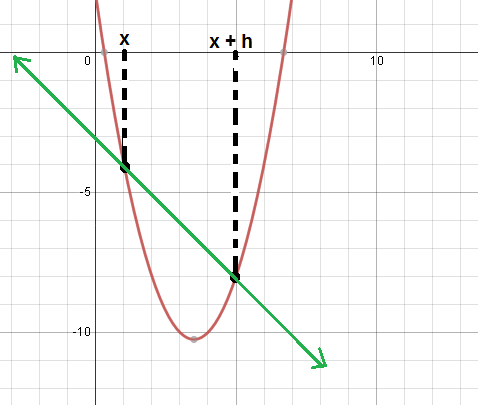# The Tangent Line Problem - Day 2 of 2

## Objective

SWBAT to use limits to find a derivative function.

#### Big Idea

The derivative function will give the slope of the tangent line for any point on f(x).

## Launch and Explore

15 minutes

Yesterday we worked on finding the slope of the tangent line at a specific point on a graph. Today our goal is to find the slope of the tangent line of any point x, that is, to find the derivative function.

We will continue working on the worksheet from yesterday and pick up on #4. Since we are trying to find the slope of the tangent line for any point x, I want to make sure that students understand that the slope will change depending on where x is located. I will sketch a few tangent lines and ask them to estimate the slopes to drive how the point that they are different. Then I will ask students what kind of answer we should get for this problem if the slopes are not all the same and hopefully they will realize that we will get an expression with x instead of a constant.

Next, I will have students recap the process of finding the slope of the tangent line at a specific point on the graph and then we will discuss together. Then we will create a diagram like the one below and talk about how to set up the limit equation to find the slope of tangent line at x.At this point I will give students about 10 minutes to work with their table groups on the algebraic portion of simplifying the slope formula for the points (x, f(x)) and (x + h, f(x + h)). As I go around I will take note of any issues that I see.

Teacher Note: One mistake that usually comes up is in the simplification of the numerator of the slope formula. Since it should be f(x + h) - f(x), students will often only subtract the first term of f(x) instead of subtracting the entire quantity. Be sure that your students are distributing that subtraction sign.

## Share

20 minutes

Once students have had time to work, I will choose a student to put their work on the document camera so that we can discuss. In this video below I touch on some essential points that I am sure to make with my students.

Once we get our answer of 2x - 7, I will choose a few x-values and plug them into this expression. For example, if x = 3, then 2(3) - 7 = -1. I choose students to interpret what -1 represents - the slope of the tangent line at x = 3. Then we will look back at the graph of f(x) and make sure this makes sense. I do this for about four more different points to really stress that this expression is giving us slopes of tangent lines. It may seem repetitive, but it is absolutely necessary!

After this work I will finally unveil the name of this new function 2x - 7; it's the derivative and is something they will become very familiar with as they progress through calculus. We fill in the box on the top of page 3 of the worksheet with the formal definition of the derivative and I will introduce the notation. For the definition, I am sure to give the algebraic limit definition, but also a description of it representing the slope of the tangent line at a specific x-value.

Below the box there is a blank. The last question I will give them says, "Use the definition above to find f'(x) if f(x) = x^3 + 4x. This will be one more example for them to practice the process of finding the derivative function.

## Summarize

15 minutes

To finish the lesson, I will give students an assignment from the textbook to summarize the important concepts that we learned today. Students will be asked to find the derivative function given f(x), and to find the slope of the tangent line given a specific x-value of the function. Students will also be asked to find the equation of a tangent line for a specific x-value of a function.